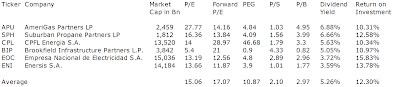## Pages

### 6 Best Yielding Utilities With A ROI Over 10 Percent

Here is a current sheet of 6 utilities with a dividend yield above 3 percent as well as a return on investment of more than 10 percent.

The return on investment is a popular performance measure and is calculated as gain from an investment less costs of the investment divided by the cost of the investment.

What is the ROI?

The average price to earnings ratio amounts to 15.06 while the forward price to earnings ratio is expected at 17.07. In average, the dividend yield amounts to 5.26 percent. Price to book ratio is 2.97 and price to sales ratio 2.10. The average ROI amounts to 12.30 percent.

Here is the table for a detailed view:Utilities With A ROI Over 10 Percent (Click to enlarge)

Related stock ticker symbols:
APU, SPH, CPL, BIP, EOC, ENI

Selected Articles: